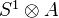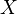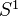# Hochschild cohomology

<]]>

<]]>
• ## Online References

Brief intro in Caldararu's notes on Derived categories of sheaves, in Homol alg folder or on arXiv.

Schuhmacher: Hochschild cohomology of complex spaces and noetherian schemes, HHA vol 6

Yekutieli: The continuous Hochschild cochain complex of a scheme, Can J Math Vol 54 (6).

<]]>
• ## Paper References

Short exposition of Hochschild cohomology (for associative algebras) in Ch 11 of Pierce (el), under Algebras.

For cohomology of algebras, see Cartan-Eilenberg, and maybe MacLane: Homology.

Course notes from Grojnowski's lectures in Cambridge.

<]]>
• ## Definition

This can be regarded as an instance of Monad cohomology.

For Hochschild cohomology of Segal cats and model cats, see Toen: Homotopical and higher categorical structures in algebraic geometry. File Toen web unpubl hab.pdf. Idea: Hochschild cohomology should be the space of endomorphisms of an identity functor.

For Hochschild cohomology of DG-categories, see Toen: Lecture on DG-categories. File Toen web unpubl swisk.pdf. Treats basic theory, localization, relation to model cats, functorial cones, K-theory and Hochschild cohomology, and descent problems.

<]]>

<]]>

<]]>

<]]>

<]]>

<]]>

<]]>
• ## Some Research Articles

K0190

arXiv:1102.5756 Cohomology of exact categories and (non-)additive sheaves from arXiv Front: math.KT by Dmitry Kaledin, Wendy Lowen We use (non-)additive sheaves to introduce an (absolute) notion of Hochschild cohomology for exact categories as Ext's in a suitable bisheaf category. We compare our approach to various definitions present in the literature.

Various things by Toen on Hochschild stuff in abstract settings.

arXiv:1001.5379 The homology of digraphs as a generalisation of Hochschild homology from arXiv Front: math.KT by Paul Turner, Emmanuel Wagner J. Przytycki has established a connection between the Hochschild homology of an algebra $A$ and the chromatic graph homology of a polygon graph with coefficients in $A$. In general the chromatic graph homology is not defined in the case where the coefficient ring is a non-commutative algebra. In this paper we define a new homology theory for directed graphs which takes coefficients in an arbitrary $A-A$ bimodule, for $A$ possibly non-commutative, which on polygons agrees with Hochschild homology through a range of dimensions.

Toen: Algebres simplicicales etc, file Toen web prepr rhamloop.pdf. Comparison between functions on derived loop spaces and de Rham theory. Take a smooth k-algebra, k aof char zero. Then (roughly) the de Rham algebra of A and the simplical algebradetermine each other (functorial equivalence). Consequence: For a smooth k-scheme, the algebraic de Rham cohomology is identified with-equivariant functions on the derived loop space of. Conjecturally this should follow from a more general comparison between functions on the derived loop space and cyclic homology. Also functorial and multiplicative versions of HKR type thms on decompositions of Hochschild cohomology, for any separated k-scheme.

Title: Operads of natural operations I: Lattice paths, braces and Hochschild cochains. Authors: Michael Batanin, Clemens Berger and Martin Markl. In this first paper of a series we study various operads of natural operations on Hochschild cochains and relationships between them. http://arxiv.org/abs/0906.4097

<]]>

<]]>